# Swift系列六 - 结构体与类的本质区别

## 一、结构体

struct Date {
var year: Int;
var month: Int;
var day: Int;
}
var date = Date(year: 2019, month: 06, day: 02)


Date(year: 2019, month: 06, day: 02)传入的是所有成员值，用来初始化所有成员（叫做存储属性）。

### 1.1. 结构体的初始化器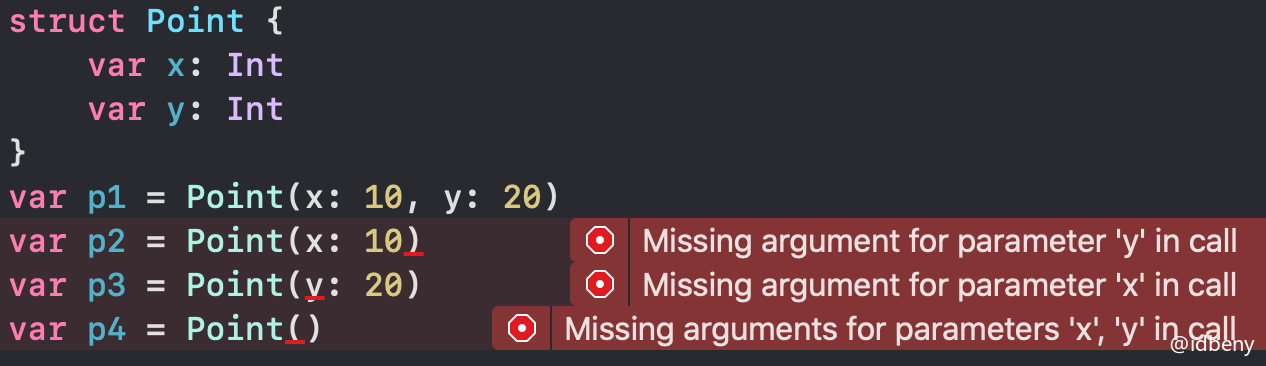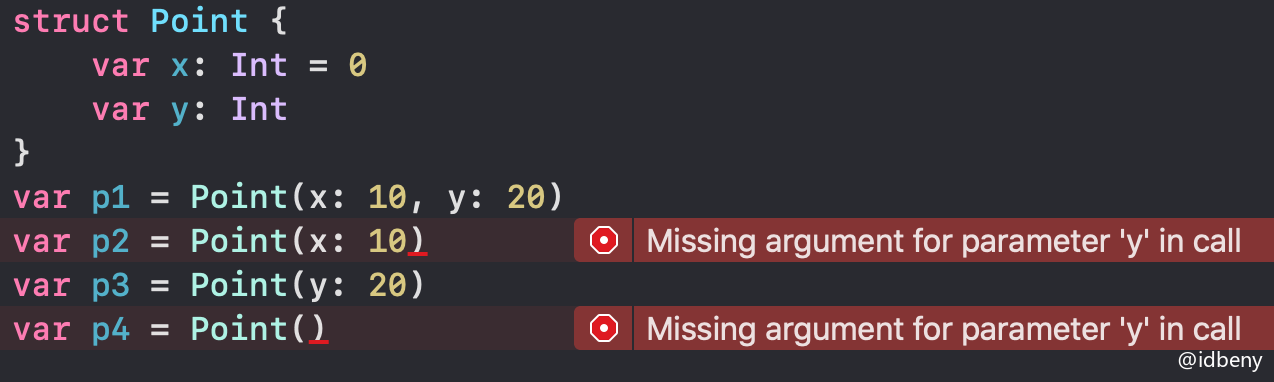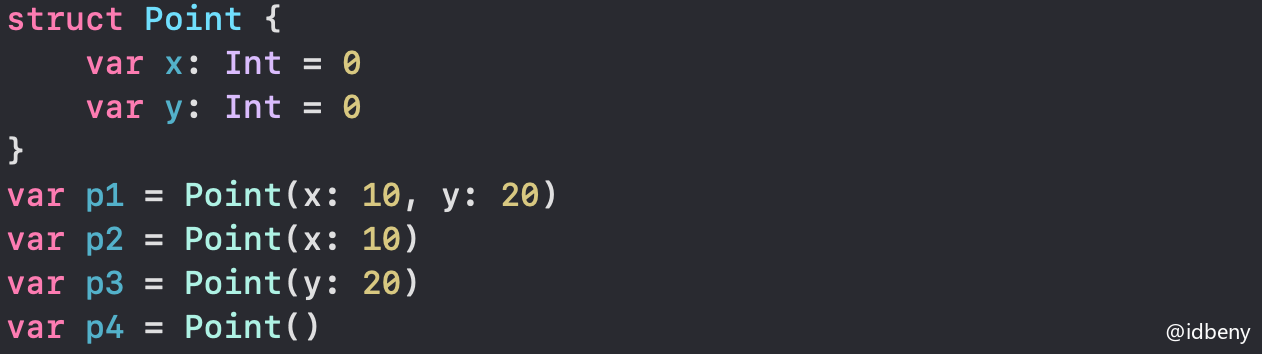> 思考：下面的代码能否编译通过？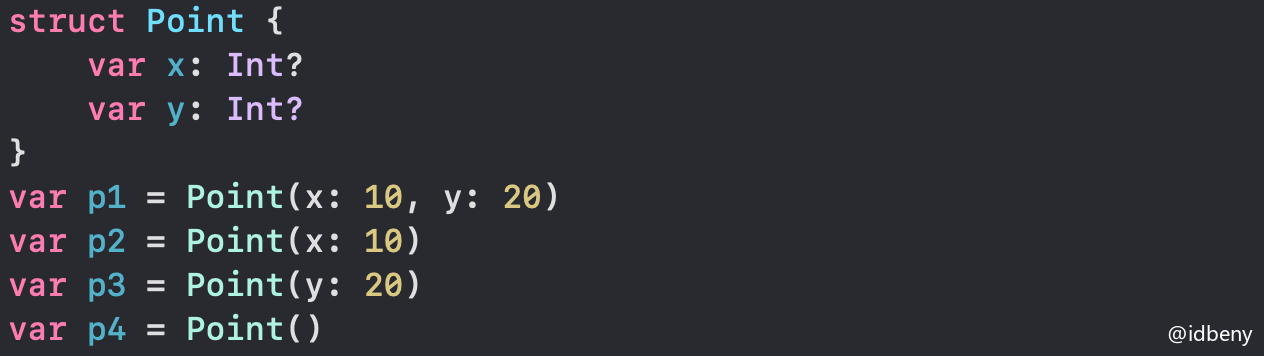### 1.2. 自定义初始化器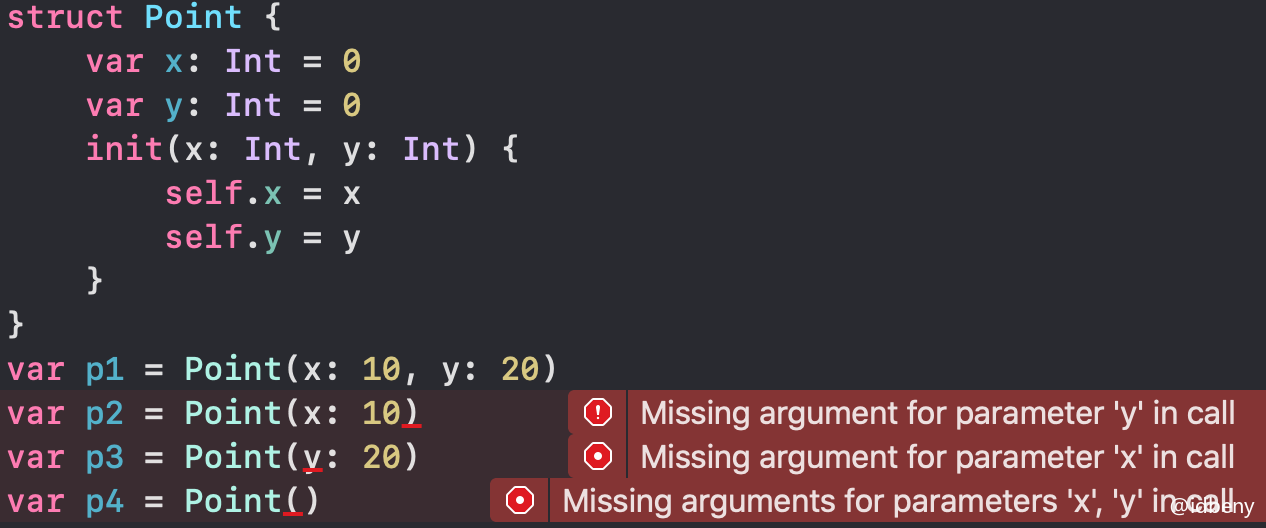### 1.3. 探究结构体初始化器的本质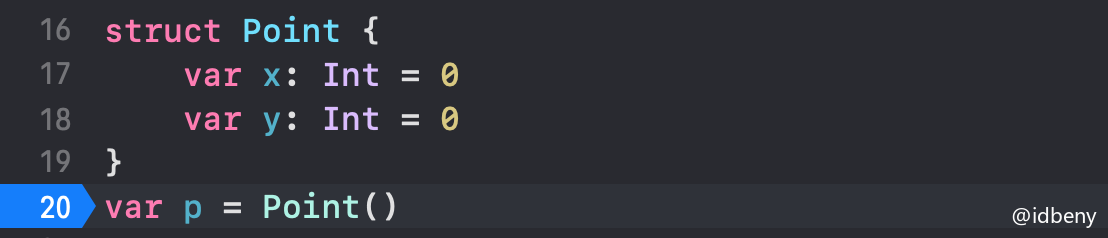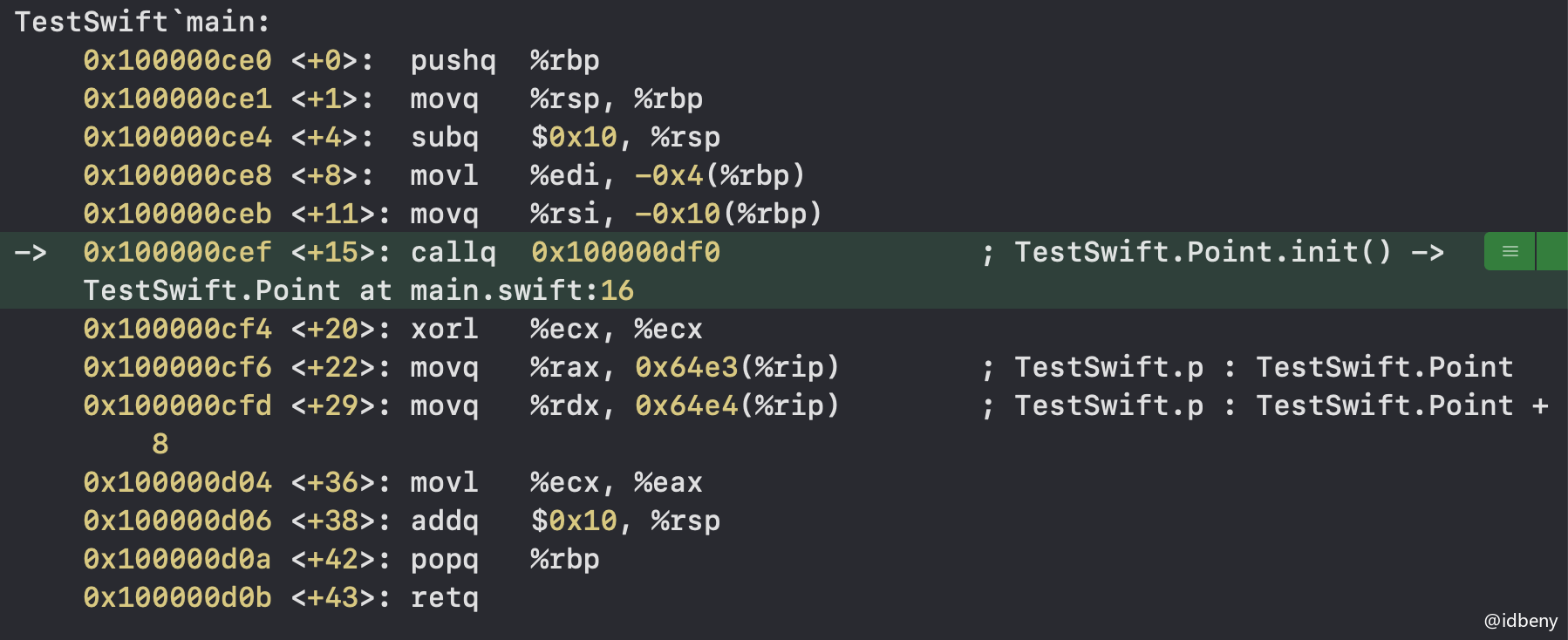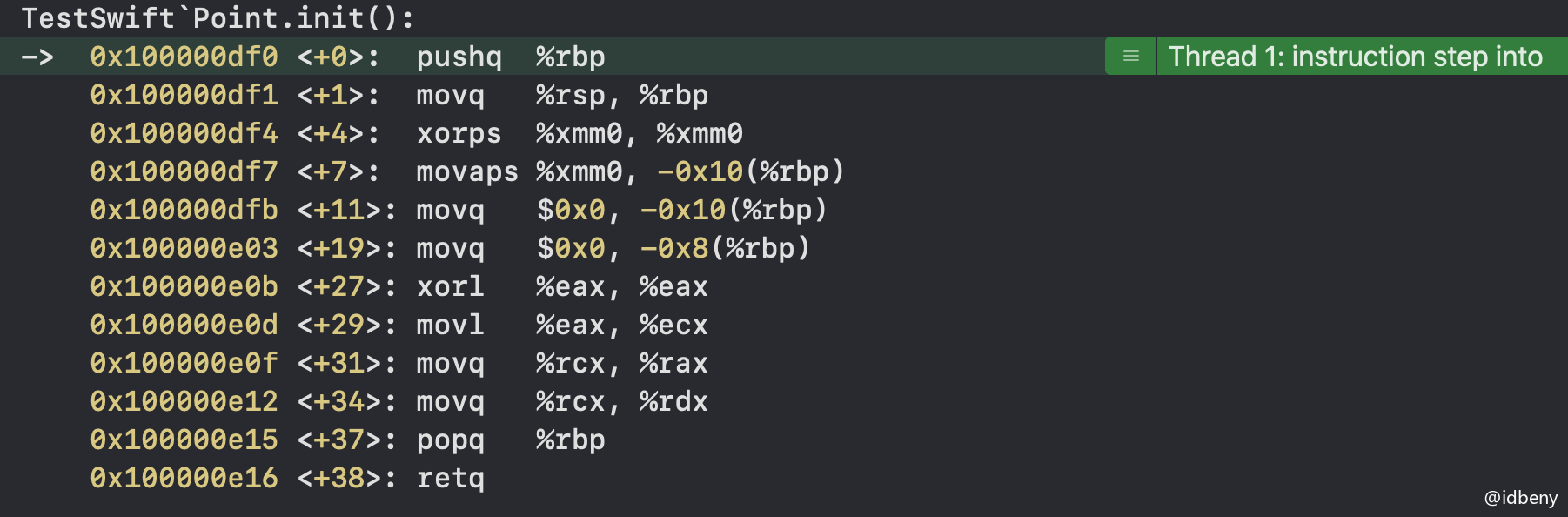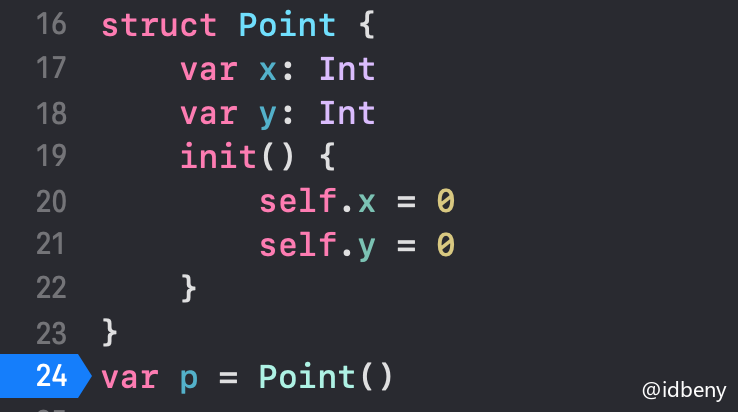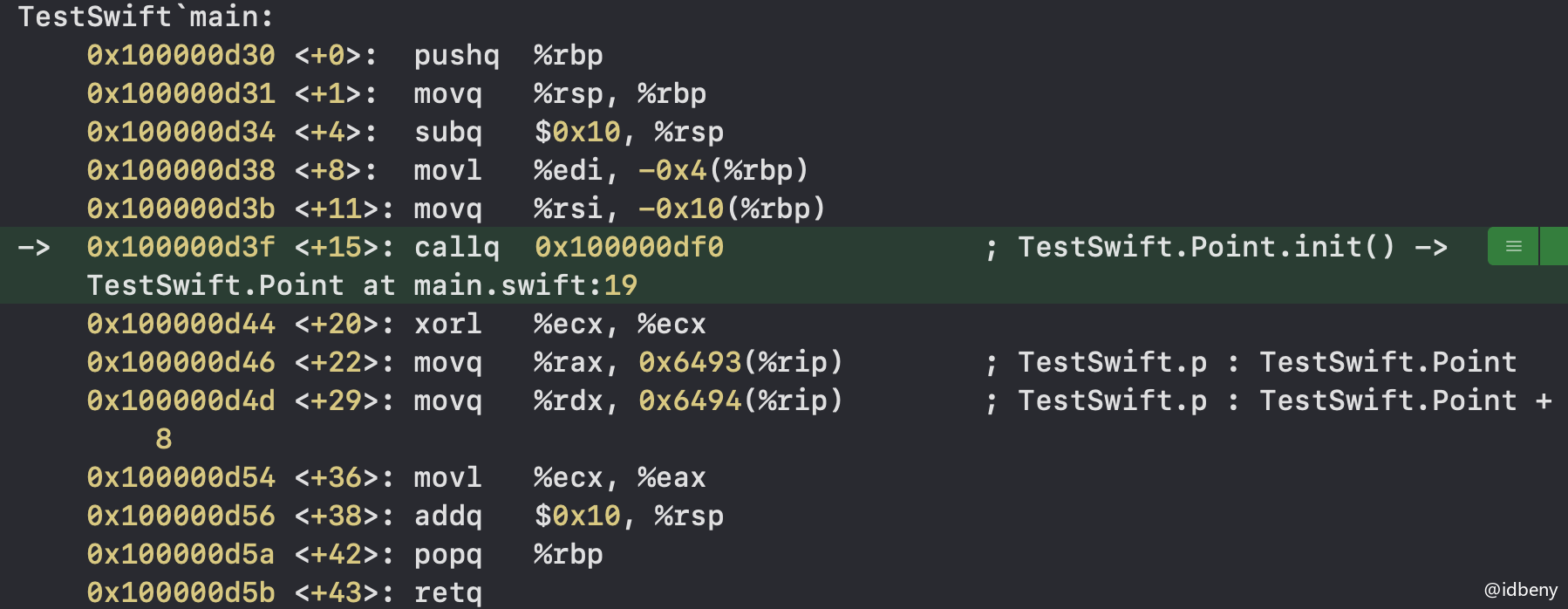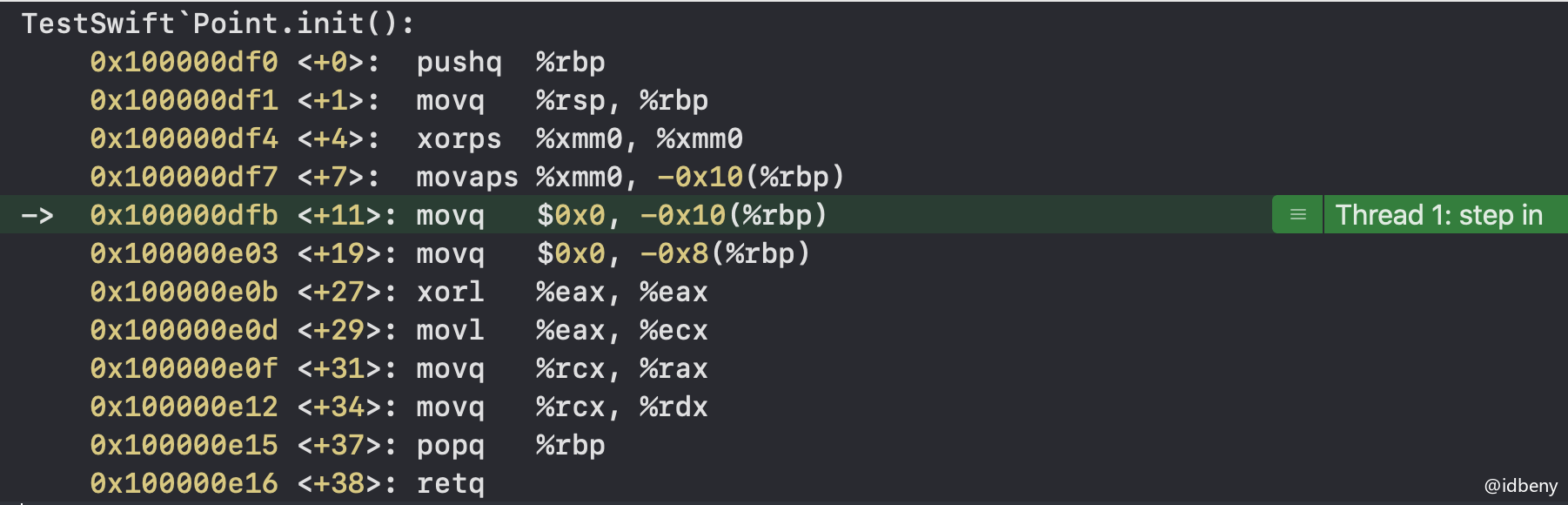### 1.4. 结构体的内存结构

struct Point {
var x = 10
var y = 20
var b = true
}
var p = Point()
print(Mems.memStr(ofVal: &amp;p))
print(MemoryLayout<point>.size)
print(MemoryLayout<point>.stride)
print(MemoryLayout<point>.alignment)

/*
输出：
0x000000000000000a 0x0000000000000014 0x0000000000000001
17
24
8
*/


## 二、类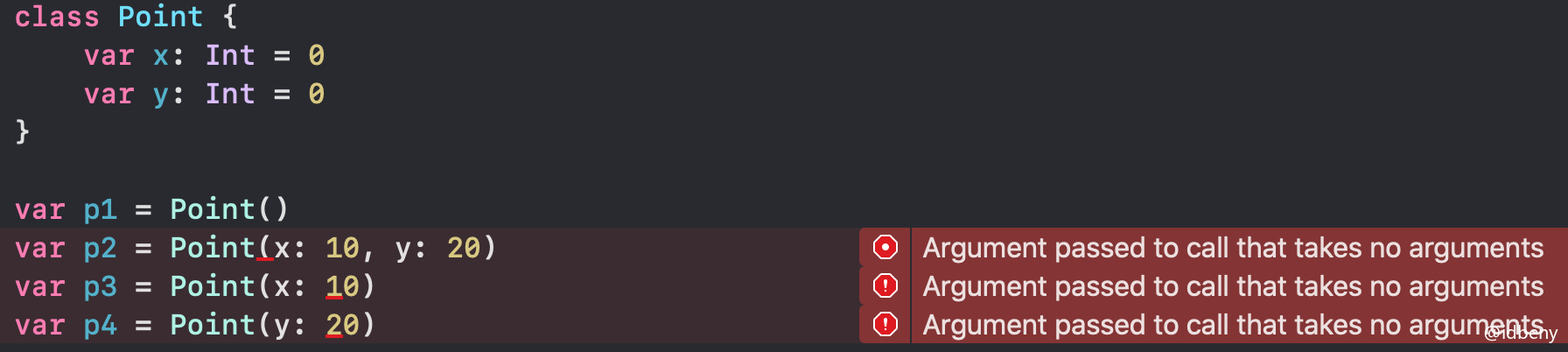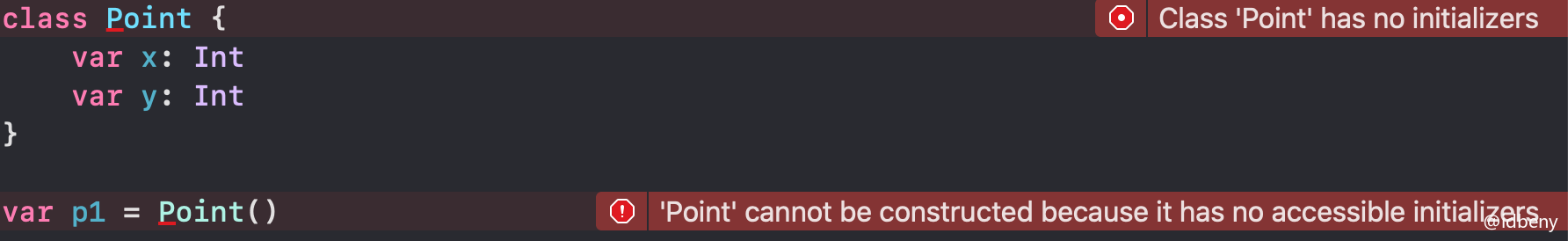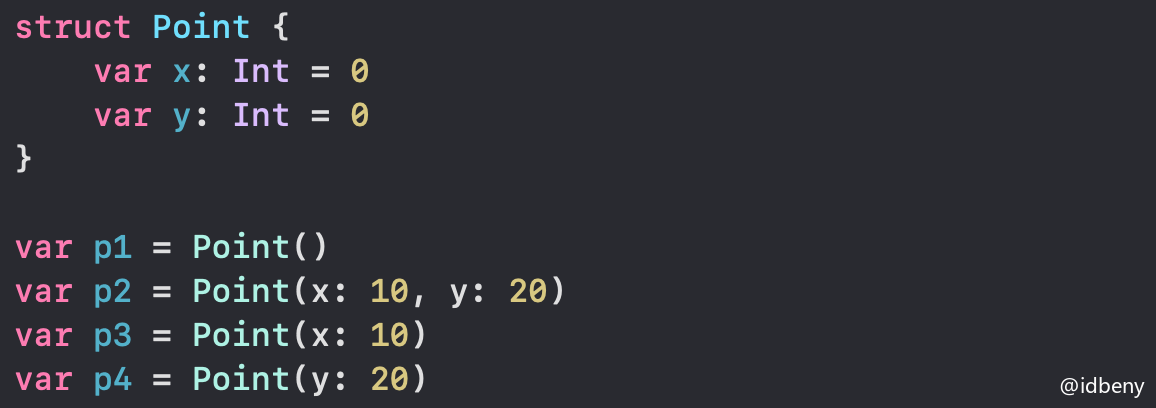### 2.1. 类的初始化器

class Point {
var x: Int = 0
var y: Int = 0
}

var p1 = Point()


class Point {
var x: Int
var y: Int
init() {
self.x = 0
self.y = 0
}
}

var p1 = Point()


## 三、结构体与类的本质区别

### 3.1. 内存分析结构体与类

class Size {
var width: Int = 1
var height: Int = 2
}

struct Point {
var x: Int = 3
var y: Int = 4
}

func test() {
var size = Size()
print("class-size对象的内存",Mems.memStr(ofRef: size))
print("class-size指针的内存地址",Mems.ptr(ofVal: &amp;size))
print("class-size对象的内存地址",Mems.ptr(ofRef: size))
print("class-size.width的内存地址",Mems.ptr(ofVal: &amp;size.width))
print("class-size.height的内存地址",Mems.ptr(ofVal: &amp;size.height))
var point = Point()
print("struct-point对象的内存",Mems.memStr(ofVal: &amp;point))
print("struct-point的内存地址",Mems.ptr(ofVal: &amp;point))
print("struct-point.x的内存地址",Mems.ptr(ofVal: &amp;point.x))
print("struct-point.y的内存地址",Mems.ptr(ofVal: &amp;point.y))
}
test()
/*
输出：
class-size对象的内存 0x00000001000092a8 0x0000000200000002 0x0000000000000001 0x0000000000000002
class-size指针的内存地址 0x00007ffeefbff4d0
class-size对象的内存地址 0x000000010061fe80
class-size.width的内存地址 0x000000010061fe90
class-size.height的内存地址 0x000000010061fe98

struct-point对象的内存 0x0000000000000003 0x0000000000000004
struct-point的内存地址 0x00007ffeefbff470
struct-point.x的内存地址 0x00007ffeefbff470
struct-point.y的内存地址 0x00007ffeefbff478
*/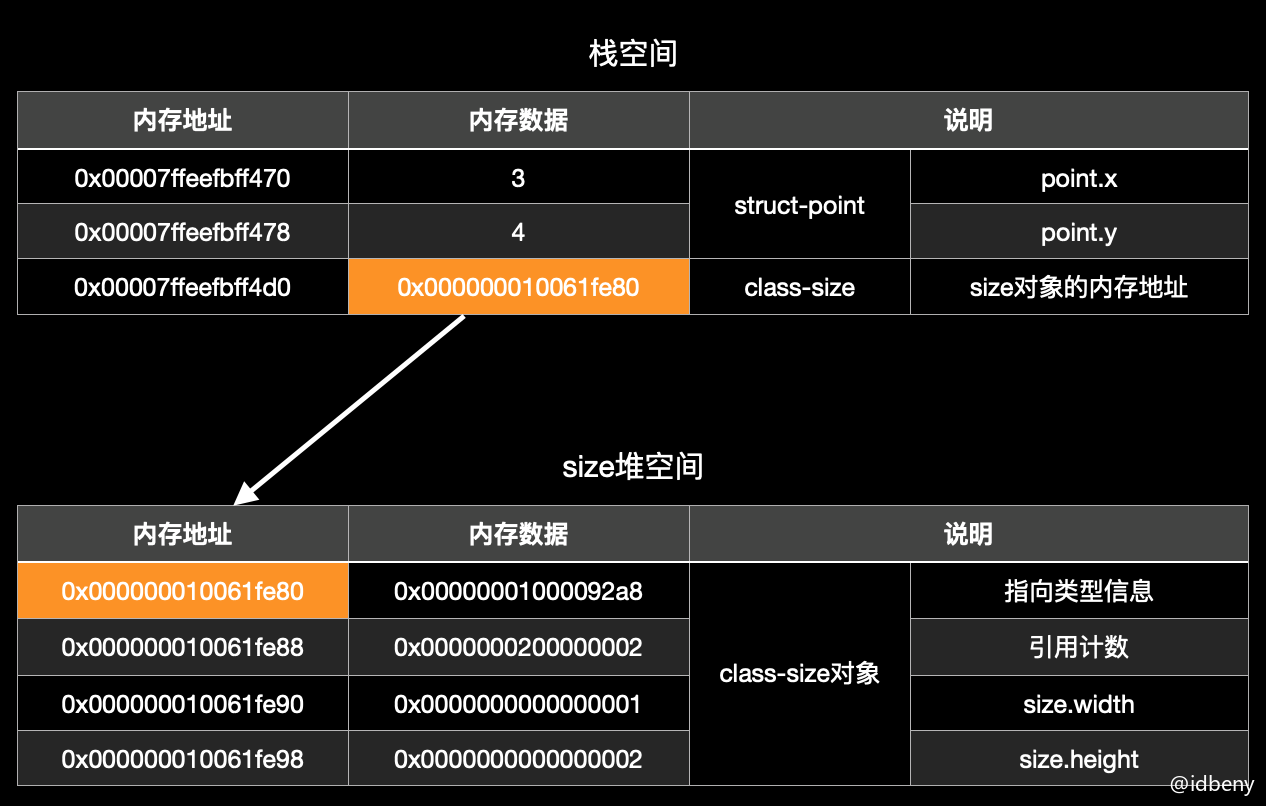> 注意：在C语言中，结构体是不能定义方法的，但是在C++Swift中，可以在结构体和类中定义方法。在64bit环境中，指针占用8个字节。
>> 扩展：值类型（结构体、枚举）的内存根据所处的位置不同，内存的位置也不一样。例如，定义一个全局的结构体，内存在数据段（全局区）中；如果在函数中定义，内存存放在栈空间；如果在类中定义一个结构体，内存跟随对象在堆空间。

### 3.2. 汇编分析结构体与类

Swift中，创建类的实例对象，要向堆空间申请内存，大概流程如下：

• Class.__allocating_init()
• libswiftCore.dylib:_swift_allocObject_
• libswiftCore.dylib:swift_slowAlloc
• libsystem_malloc.dylib:malloc

import Foundation

class Point {
var x: Int = 3
var y: Int = 4
var b: Bool = true
}
var p = Point()
print(class_getInstanceSize(type(of: p)))
print(class_getInstanceSize(Point.self))
/*
输出：
40
40
*/


> 扩展：如果底层调用了alloc或malloc函数，说明该对象存在堆空间，否则就是在栈空间。

#### 3.2.1. 汇编分析结构体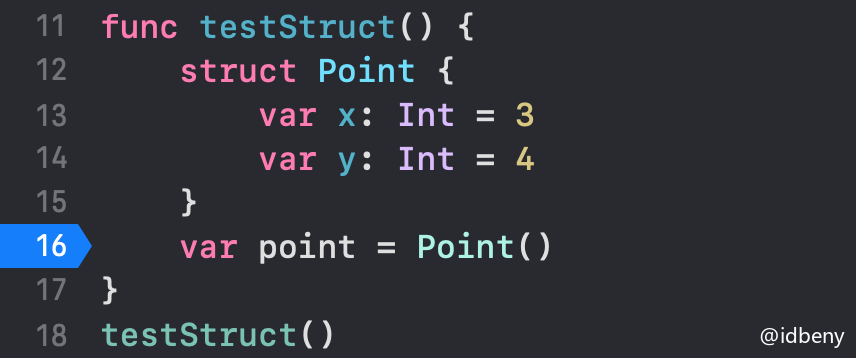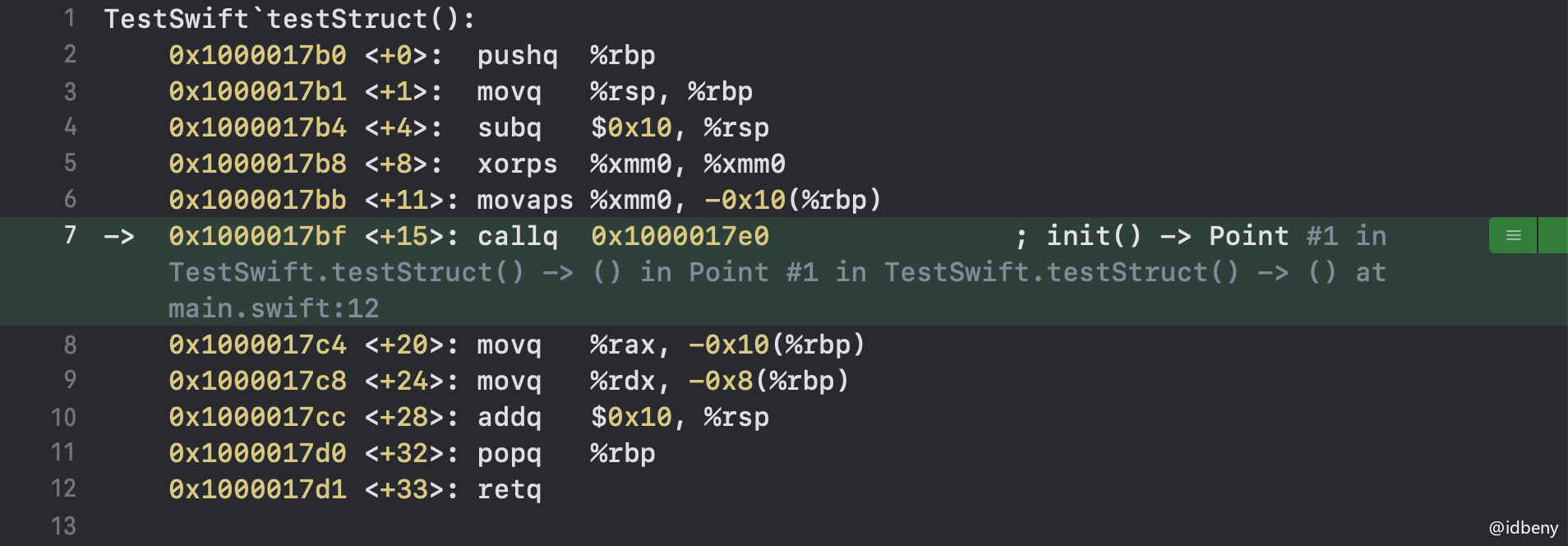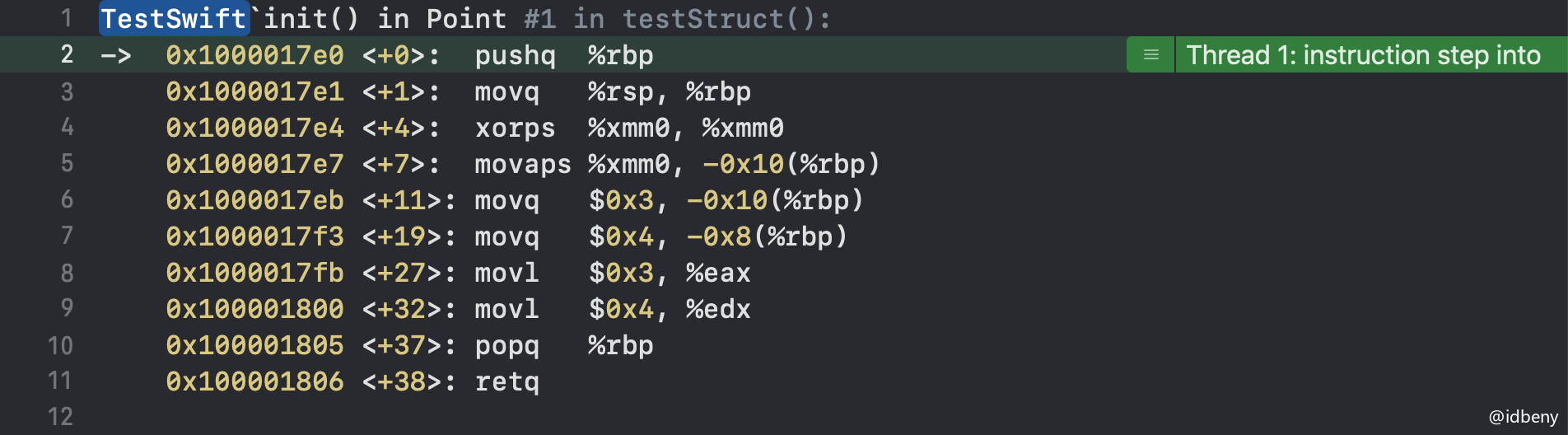> 扩展：一般情况下，rbp就是局部变量，rip是全局变量，ret是函数返回。

#### 3.2.2. 汇编分析类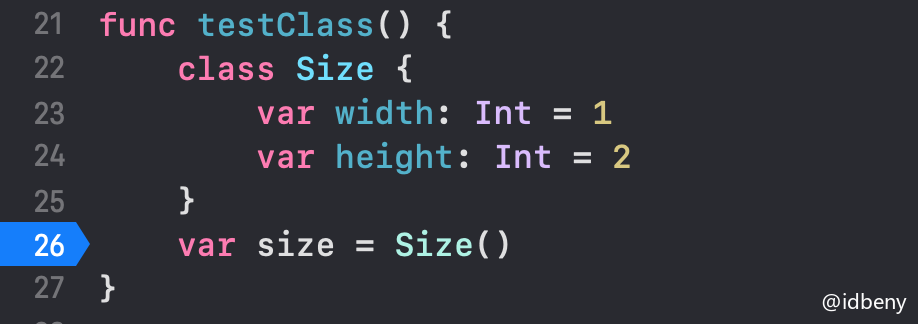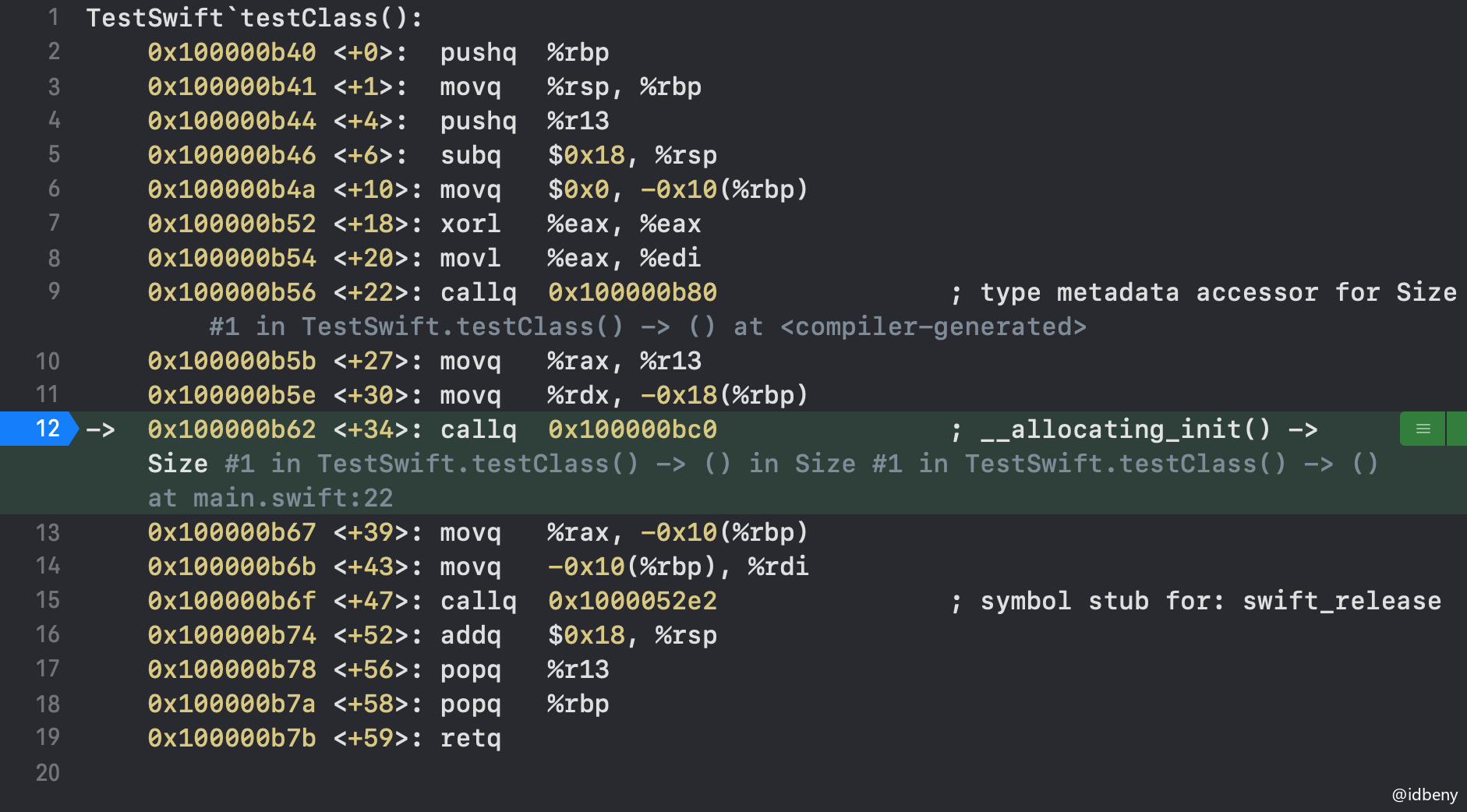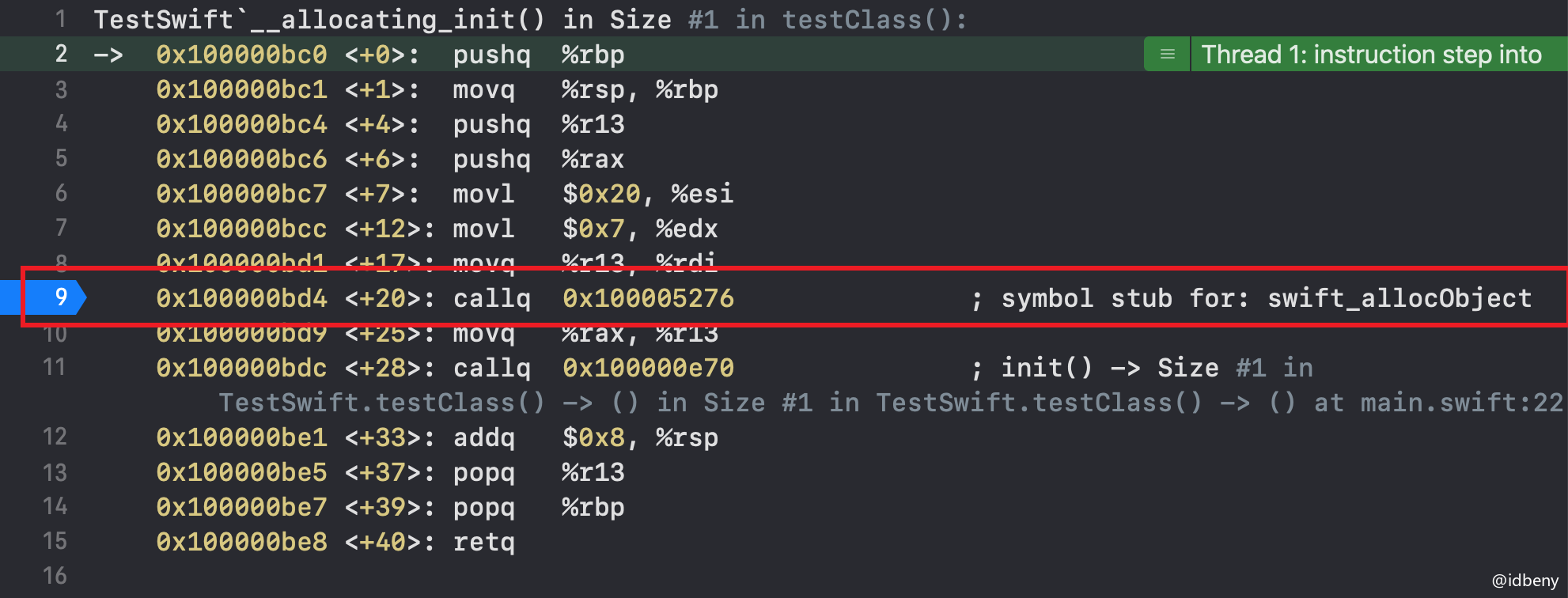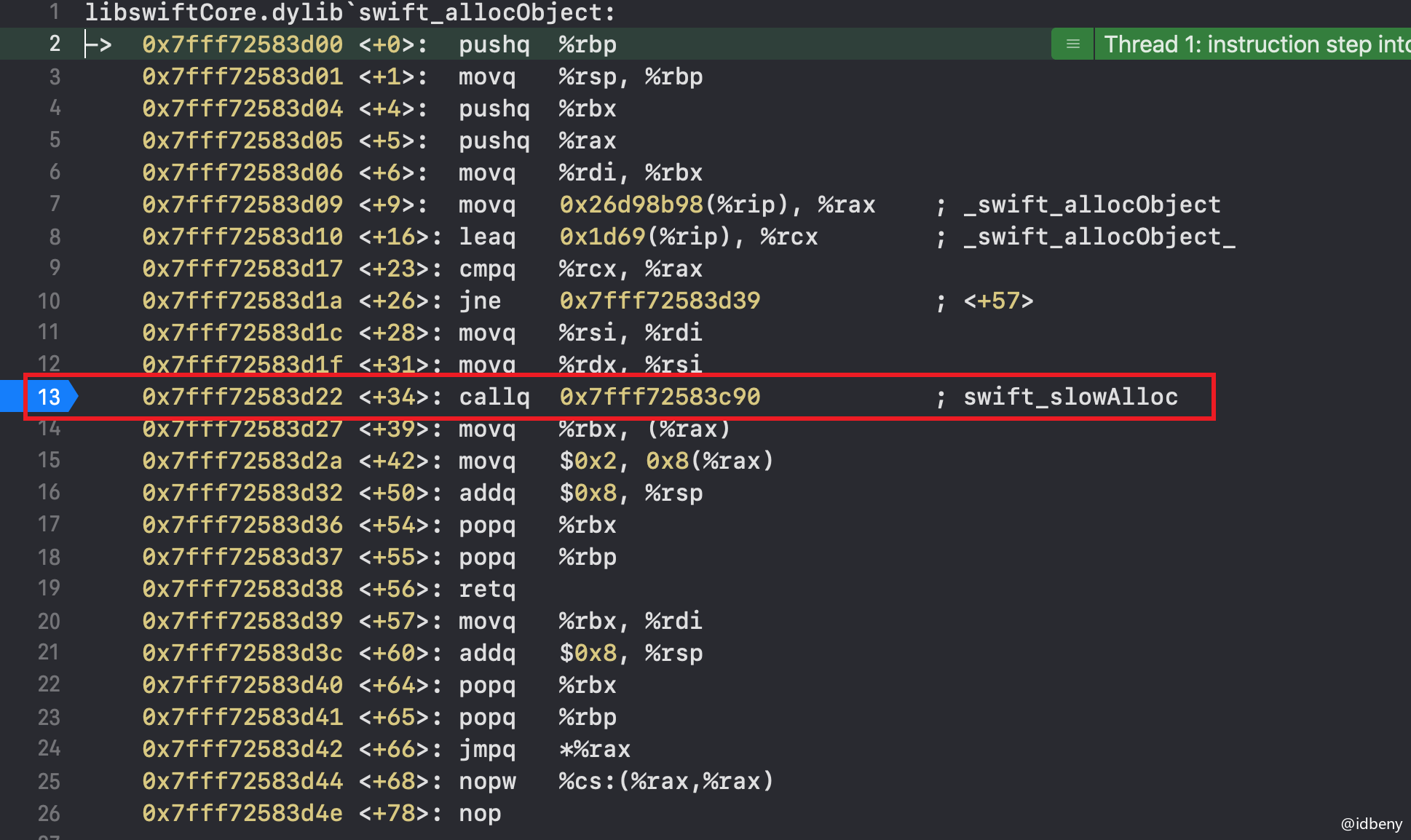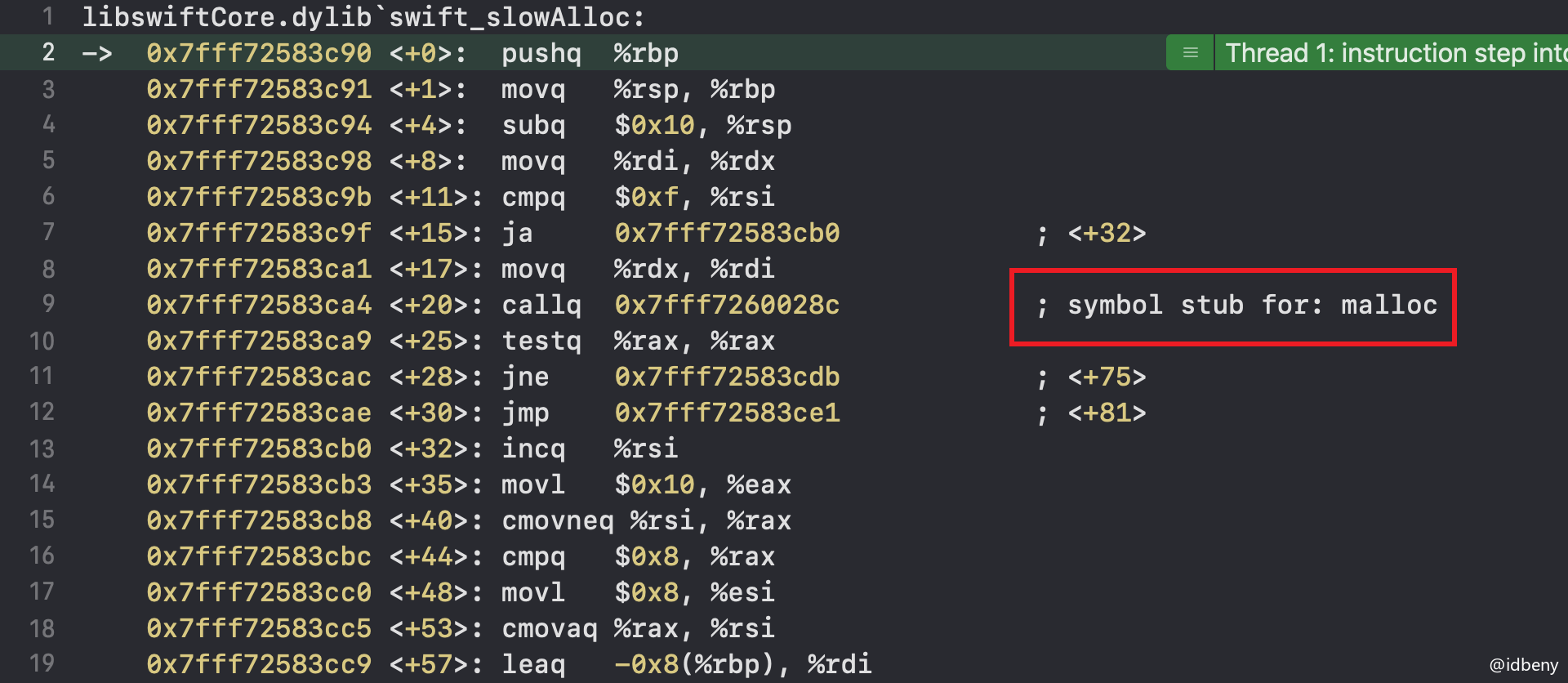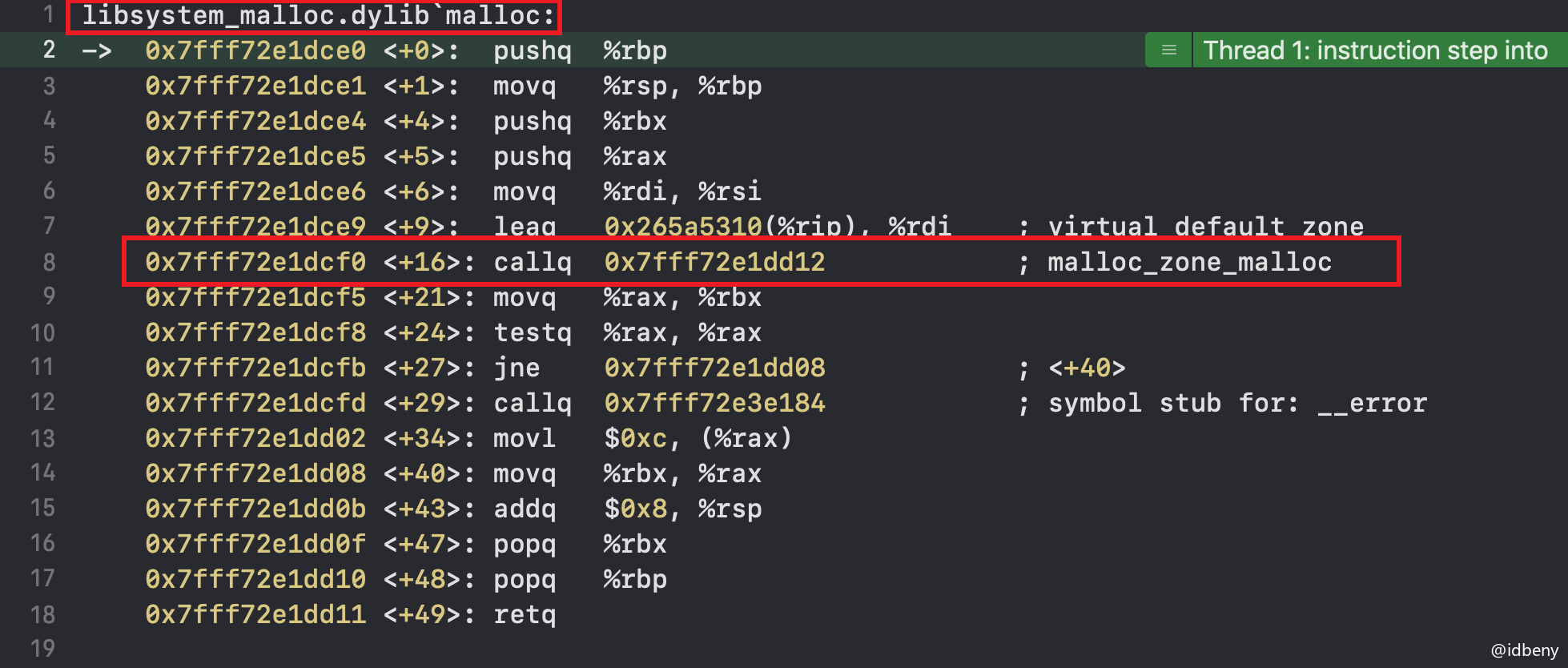posted @ 2021-05-08 16:29  1024星球  阅读(100)  评论(0编辑  收藏  举报